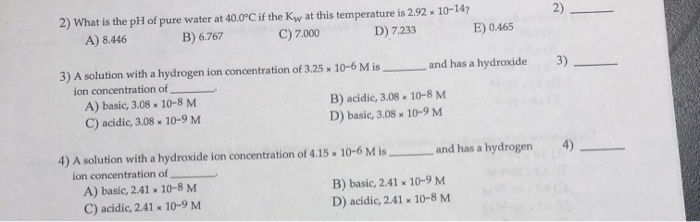# What is the ph of pure water at 40.0°c if the kw at this temperature is 2.92 × 10-14?2) What is the pH of pure water at 40.0°C if the Kw at this temperature is 2.92 x 10-14? 2) C) 7.000 A) 8.446 B) 6.767 D) 7.233 E) 0.465 3) A solution with a hydrogen ion concentration of 3.25 x 10-6 M is and has a hydroxide ion concentration of A) basic, 3.08x 10-8 M B) acidic, 3.08 D) basic, 3.08 10-8 M C) acidic, 3.08 10-9 M 10-9 M 4) A solution with a hydroxide ion concentration of 4.15 10-6 M is and has a hydrogen ion concentration of A) basic, 2.41 x 10-8 M C) acidic, 2.41 x 10-9 M B) basic, 2.41x 10-9 M D) acidic, 2.41 x 10-8 M

​​​​​​Formulas to be used:
[H+] *[OH-] = Kw
pH = – log [H+]
And
[H+] = [OH-] ( note : this formula is
valid only for pure water)
In general Kw = 10-14 (unless specified this is
always used)
2)B) 6.767
Explaination:
Given : pure water and Kw = 2.92 x 10-14
[H+] *[OH-] = Kw
(Pure water ,so [H+] = [OH-] )
[H+] *[H+] = Kw
[H+]2 = Kw
[H+]2 = 2.92 x 10-14
[H+] = (2.92 x 10-14 )1/2
[H+] =1.7088 x 10-7
pH = -log[H+]
pH = -log(1.7088 x 10-7)
pH = 6.767
3)C)acidic ,3.08 x 10-9M
Explaination:
Given : [H+] =3.25 x 10-6M
For calculation of pH :
pH = -log[H+]
pH = -log (3.25 x 10-6)
pH= 5.48
pH<7 , therefore , acidic For [OH-] calculation: [H+] *[OH-] = Kw (3.25 x 10-6)*[OH-] = 10-14 [OH-] = (10-14 )/(3.25 x 10-6) [OH-] = 3.08 x 10-9M 4) B) basic , 2.41 x 10-9 Explaination: Given : [OH-] = 4.15 x 10-6M For calculation of [H+] : [H+] *[OH-] = Kw [H+] *(4.15 x 10-6) = 10-14 [H+] = (10-14 )/(4.15 x 10-6) [H+] = 0.2409 x 10-8 [H+] = 2.41 x 10-9M For pH calculation : pH = -log[H+] pH = - log(2.41 x 10-9) pH = 8.61 pH>7 , therefore , basic